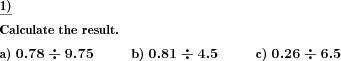Custom math worksheets at your fingertips# Details for problem "Decimal fraction division"

Quickname: 8100

Elementary School, Primary School, Junior High School, Middle School, High School.

## Summary

A decimal fraction has to be divided by another decimal fraction.

## Example## Description

Two decimal fractions are given, one has to be divided by the other.

The number of places before the decimal point and the number of decimals can be selected individually for the dividend and for the divisor. Accordingly, the decimal places of the quotient will be determined by these selections for the dividend and divisor. It may also happen that the quotient turns out to be a whole number.

The number of problems can be selected.

Download free printable worksheets for this math problem here. The worksheet contains the problems only, the solution sheet includes the answers. Just click on the respective link.

•Worksheet 1Solution sheet with answers
•Worksheet 2Solution sheet with answers
•Worksheet 3Solution sheet with answers

If you can not see the solution sheets for download, they may be filtered out by an ad blocker that you may have installed. If this is the case, please allow ads for this page and reload the page. The solution sheets will then reappear.

• Do these sample worksheets do not really fit?
• Do you need more math worksheets, with a different level of difficulty?
• Would you like to combine different problems on a worksheet and adjust them to your needs?
• As a teacher, you can put together your own worksheets using the automatically generated math problems provided.
With a free initial credit, you can start creating your own math worksheets in a few minutes.

You can try it for free! Register here, to create custom worksheets now!

## Customization options for this problem

Parameter
Possible values
Number of problems
1, 2, 3, 4, 5, 6, 7, 8, 9, 10
Places dividend
0, 1, 2, 3
Decimals dividend
1, 2, 3, 4
Places divisor
0, 1, 2, 3
Decimals divisor
1, 2, 3, 4

## Similar problems

Remark
Description
Divisor is a power of ten
A decimal fraction has to be divided by a power of ten.
Divisor is a whole number
A decimal fraction has to be divided by a whole number.
Special case: Halving a number
Divide numbers by two, whole numbers or with decimal places.Deutsche Version dieser Aufgabe
These informational pages with samples describe math problems that can be combined on custom math worksheets with solutions for home and K-12 school use.
Deutsche Seiten
×